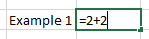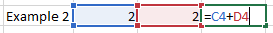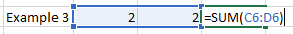Classes available in-person (strict social distancing) or live online with an extended free retake period. See details.# Why Excel is Better than a Calculator

In this article, we'll go through the basics of Excel calculations and learn why Excel serves us better than using a calculator.

### Excel as a Calculator

Let's go through some basic examples for doing calculations in Excel. Think of Excel as a calculator. If we wanted to do a simple calculation like 2+2 we could go ahead to a cell in Excel and type “=2+2” and hit enter and we’ll get the correct answer.Note, you must always begin formulas in Excel with an equals sign. The way we did that calculation is the way we would type things into a calculator, but that is generally not the best practice for using Excel. What we’ll want to do in this case is put the number 2 in two different cells and then we have a couple of options to do our calculation. First, we can go to a new cell and click = then scroll over the first cell with the 2 in it and then hit + and then go to the cell with the other 2 and then hit enter.The second option to do this is to go to a new cell and then hit = and then type “sum(“ and then highlight the two cells with the values in there and then hit enter. We can either do one cell plus the other or we can use the SUM function to add up both cells. Both options will give us the correct answer.### The Dynamic Nature of Excel

The reason why these are both better solutions than the traditional calculator route is that this allows us to have a dynamic formula. When we type the value into two different cells and then have another cell with the answer we can then easily change the values in the cells and Excel will automatically recalculate the solution for us. While this is a very simple example, when we are doing more complex things in Excel like managing budgets or financial modeling, we will need our formulas to work accordingly to fully leverage Excel.

We can also apply this logic to multiplication, division, and more complex calculations that we’ll cover in later articles. If we have a list of products with their quantities and prices we can very easily get the total value of all our products by multiplying the quantity by the price for the first value and then drag down the formula for Excel to automatically update the formula for the rest of the list. This is another benefit of having formulas set up the right way in Excel.

Some of the other basic calculation functions that we’ll cover in our Introduction to Excel include:

• SUM - the sum function allows us to easily add up a whole list of numbers to get the total

• AVERAGE - this function gives us the average of a list of numbers

• MEDIAN - find the median in a list of numbers